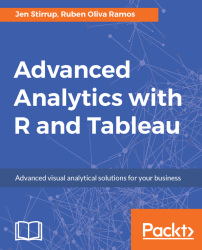•#### Advanced Analytics with R and Tableau#### Overview of this book

Tableau and R offer accessible analytics by allowing a combination of easy-to-use data visualization along with industry-standard, robust statistical computation. Moving from data visualization into deeper, more advanced analytics? This book will intensify data skills for data viz-savvy users who want to move into analytics and data science in order to enhance their businesses by harnessing the analytical power of R and the stunning visualization capabilities of Tableau. Readers will come across a wide range of machine learning algorithms and learn how descriptive, prescriptive, predictive, and visually appealing analytical solutions can be designed with R and Tableau. In order to maximize learning, hands-on examples will ease the transition from being a data-savvy user to a data analyst using sound statistical tools to perform advanced analytics. By the end of this book, you will get to grips with advanced calculations in R and Tableau for analytics and prediction with the help of use cases and hands-on examples.
Advanced Analytics with R and TableauCreditswww.PacktPub.comCustomer FeedbackPrefaceFree Chapter
Advanced Analytics with R and TableauThe Power of RA Methodology for Advanced Analytics Using Tableau and RPrediction with R and Tableau Using RegressionClassifying Data with TableauIndex## Getting started with regression

Regression means the unbiased prediction of the conditional expected value, using independent variables, and the dependent variable. A dependent variable is the variable that we want to predict. Examples of a dependent variable could be a number such as price, sales, or weight. An independent variable is a characteristic, or feature, that helps to determine the dependent variable. So, for example, the independent variable of weight could help to determine the dependent variable of weight.

Regression analysis can be used in forecasting, time series modeling, and cause and effect relationships.

### Simple linear regression

R can help us to build prediction stories with Tableau. Linear regression is a great starting place when you want to predict a number, such as profit, cost, or sales. In simple linear regression, there is only one independent variable x, which predicts a dependent value, y.

Simple linear regression is usually expressed with a line that identifies...